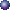# Visual Algebra

### 1. Functions and Graphs

#### Definitions of function, domain, rangeMapping as Function: exampleGraph as Function: exampleDomain of a Function: exampleRange of a Function: example

#### Graphing TechniquesHorizontal Translation: example1, example2Vertical Translation: example1, example2Reflection about x-axis: exampleReflection about y-axis: exampleAbsolute Value: example

#### Asymptotes:Vertical: exampleHorizontal: exampleOblique: example